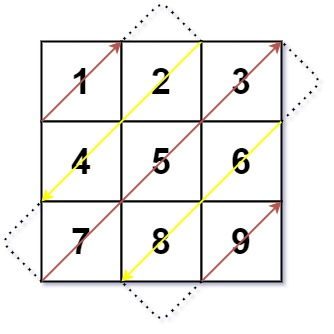0498. 对角线遍历

# 0498. 对角线遍历#

• 标签：数组、矩阵、模拟
• 难度：中等

## 题目大意 #

• $m == mat.length$。
• $n == mat[i].length$。
• $1 \le m, n \le 10^4$。
• $1 \le m * n \le 10^4$。
• $-10^5 \le mat[i][j] \le 10^5$。

• 示例 1：
 1 2  输入：mat = [[1,2,3],[4,5,6],[7,8,9]] 输出：[1,2,4,7,5,3,6,8,9]## 解题思路 #

### 思路 1：找规律 + 考虑边界问题 #

1. 当「行号 + 列号」为偶数时，遍历方向为从左下到右上。可以记为右上方向 (-1, +1)，即行号减 1，列号加 1
2. 当「行号 + 列号」为奇数时，遍历方向为从右上到左下。可以记为左下方向 (+1, -1)，即行号加 1，列号减 1

1. 向右上方向移动时：
1. 如果在最后一列，则向下方移动，即 x += 1
2. 如果在第一行，则向右方移动，即 y += 1
3. 其余情况想右上方向移动，即 x -= 1y += 1
2. 向左下方向移动时：
1. 如果在最后一行，则向右方移动，即 y += 1
2. 如果在第一列，则向下方移动，即 x += 1
3. 其余情况向左下方向移动，即 x += 1y -= 1

### 思路 1：代码 #

  1 2 3 4 5 6 7 8 9 10 11 12 13 14 15 16 17 18 19 20 21 22 23 24 25 26 27 28 29 30 31 32 33 34  class Solution: def findDiagonalOrder(self, mat: List[List[int]]) -> List[int]: rows = len(mat) cols = len(mat) count = rows * cols x, y = 0, 0 ans = [] for i in range(count): ans.append(mat[x][y]) if (x + y) % 2 == 0: # 最后一列 if y == cols - 1: x += 1 # 第一行 elif x == 0: y += 1 # 右上方向 else: x -= 1 y += 1 else: # 最后一行 if x == rows - 1: y += 1 # 第一列 elif y == 0: x += 1 # 左下方向 else: x += 1 y -= 1 return ans 

### 思路 1：复杂度分析 #

• 时间复杂度：$O(m \times n)$。其中 $m$、$n$ 分别为二维矩阵的行数、列数。
• 空间复杂度：$O(m * n)$。如果算上答案数组的空间占用，则空间复杂度为 $O(m * n)$。不算上则空间复杂度为 $O(1)$。